﻿ 人工智能在水文预报中的应用研究

# 人工智能在水文预报中的应用研究Hydrological Forecasting Using Artificial Intelligence Techniques

Abstract: The key techniques and bottlenecks of artificial intelligences in data-driven hydrological model were reviewed thoroughly. Gamma test method was used to optimize the input combination of data-driven model to reduce the white noisy error. The machine learning techniques, such as batch-size learning, regularization and drop out neuron were incorporated into a long-short-term memory (LSTM) deep learning neural network to simulate nonlinear, stochastic and non-static processes in hydrological forecast under changing environment. The application results in study area between Xiangjiaba and Three Gorges Reservoir inter-basin indicate that the forecasting accuracy from one to three days lead-time reaches A-grade (reliability ≥ 85%) and is improved effectively by integrating LSTM neural network and three deep learning auxiliary algorithms in the interests of conquering model over parameterization and overfitting bottlenecks.

1. 引言

2. 研究内容及关键技术

2.1. 数据输入选择

Gamma Test由Stefánsson等人于1997年提出，它是一个独立于模型的数据分析方法，属于资料导向的统计分析方法，可估计资料中包含噪声的程度，具有良好的实用性  。Gamma Test具有以下三个功能：1) 确定训练出最优数据驱动模型的最小数据集；2) 时间上：依据洪水传播时间，确定输入变量的最优时滞；3) 空间上：确定输入变量最优组合。由于执行一次Gamma test耗时短，因此可将众多可能输入因子同时放入，评估所有可能输入组合产生的噪声估计值，进而有效地选择产生噪声最小的输入因子组合作为后续构建模型的输入项。考虑到流域内点降雨变量数目众多，且以一对一的相关系数统计分析无法全面挖掘出各类模型输入变量组合的相关强度，而运用Gamma Test则可有效地找出最适合推估降雨-径流映射的因子组合。Gamma Test基本原理和计算步骤，详见文献   。

2.2. 静态与动态神经网络

2.3. 长短期记忆神经网络(LSTM)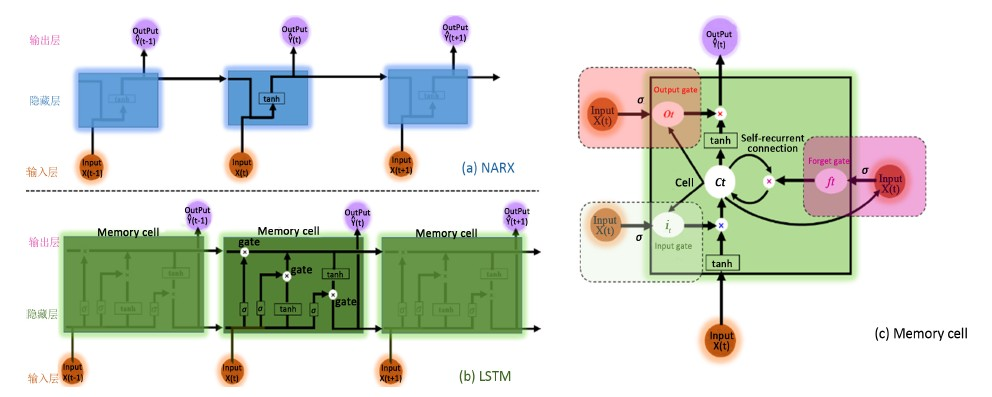Figure 1. Comparison analysis between NARX and LSTM neural network architectures

1) Layer 1：记忆输入层(Input layer)

${\stackrel{˜}{C}}_{t-1}=\mathrm{tanh}\left({W}_{c}{X}_{t}+{U}_{c}{h}_{t-1}+{b}_{c}\right)$ (1)

2) Layer 2：隐藏层(Hidden layer)

① 输入门(Input gate)：在产生新记忆之前，网络需要判定当前信息 ${X}_{t}$ 重不重要，并做出是否记忆当前某些信息的决策。输入门根据输入信息和过去隐藏层状态 ${h}_{t-1}$ 共同判定记忆输入值是否值得保留，从而判定它以何种程度参与新的记忆。因此，它可以作为当前信息更新的一个评估指标。

${i}_{t}=\sigma \left({W}_{i}{X}_{t}+{U}_{i}{h}_{t-1}+{b}_{i}\right)$ (2)

② 忘记门(Forget gate)：这个门和输入门类似，但它不能决定当前输入信息是否有效，仅对过去记忆单元信息做出评估，评估出是否选择忘记过去某些信息。因此，它可以作为过去记忆单元信息的一个评估指标。

${f}_{t}=\sigma \left({W}_{f}{X}_{t}+{U}_{f}{h}_{t-1}+{b}_{f}\right)$ (3)

③ 内部回馈连结(Self-recurrent connection)：回馈连结会根据遗忘门 ${f}_{t}$ 的评估结果，合理地选择忘记部分过去的记忆，再根据输入门 ${i}_{t}$ 的评估结果，产生新记忆 ${C}_{t-1}$ 。内部回馈连结可将过去和当前的记忆信息合并后产生新记忆 ${C}_{t}$

${C}_{t}={i}_{t}\ast {\stackrel{˜}{C}}_{t}+{f}_{t}\ast {C}_{t-1}$ (4)

④ 输出门(Output gate)：它的目的是从隐藏层状态分离最终的记忆。最终记忆 ${C}_{t}$ 包含了大量不需要保存在隐藏层状态 ${h}_{t}$ 的信息，这个门能够评估出关于记忆 ${C}_{t}$ 哪些部分需要显示在隐藏层状态 ${h}_{t}$ 中。用于评估这部分信息的中间信号叫做 ${o}_{t}$ ，它和 $\mathrm{tanh}\left({C}_{t}\right)$ 的乘积组成了最后的隐藏层状态 ${h}_{t}$

${o}_{t}=\sigma \left({W}_{o}{X}_{t}+{U}_{o}{h}_{t-1}+{V}_{o}{C}_{t}+{b}_{o}\right)$ (5a)

${h}_{t}={o}_{t}\ast \mathrm{tanh}\left({C}_{t}\right)$ (5b)

3) Layer 3：输出层(Output layer)

$\stackrel{^}{Y}\left(t\right)={W}_{y}{h}_{t}+{b}_{y}$ (6)

2.4. 机器学习算法

1) 最小批量梯度下降法

2) 正则化

$L={L}_{0}+\frac{\lambda }{2N}{\sum }_{j=1}^{N}{w}_{j}^{2}$ (7)

$\frac{\partial L}{\partial w}=\frac{\partial {L}_{0}}{\partial w}+\frac{\lambda }{N}w$ (8a)

$\frac{\partial L}{\partial b}=\frac{\partial {L}_{0}}{\partial b}$ (8b)

$w\to w-\eta \left(\frac{\partial {L}_{0}}{\partial w}+\frac{\lambda }{N}w\right)$ (9a)

$w-\eta \left(\frac{\partial {L}_{0}}{\partial w}+\frac{\lambda }{N}w\right)=\left(1-\eta \frac{\lambda }{N}\right)w-\eta \frac{\partial {L}_{0}}{\partial w}$ (9b)

3) 筛选神经元

2.5. 水文预报的评估指标

1) 合格率(Reliability, R)

$R=\frac{{\sum }_{t=1}^{N}{m}_{t}}{N}×100%$ (10a)

${m}_{t}=\left\{\begin{array}{l}1,\text{\hspace{0.17em}}\text{\hspace{0.17em}}\text{\hspace{0.17em}}\text{if}\text{\hspace{0.17em}}\left(R{E}_{t}\le 20%\right)\\ 0,\text{\hspace{0.17em}}\text{\hspace{0.17em}}\text{\hspace{0.17em}}\text{\hspace{0.17em}}\text{\hspace{0.17em}}\text{\hspace{0.17em}}\text{\hspace{0.17em}}\text{\hspace{0.17em}}\text{else}\end{array}$ (10b)

$R{E}_{t}=\frac{|{Y}_{f}\left(t\right)-{Y}_{o}\left(t\right)|}{{Y}_{o}\left(t\right)}×100%$ (10c)

2) 洪峰相对误差(Relative Error of peak, REp)

REp值若大于0则代表模型洪峰预报值偏小，若小于0则代表洪峰预报值偏大，若等于0则代表无预报误差。

$R{E}_{p}=\frac{{Y}_{p}-{\stackrel{^}{Y}}_{p}}{{Y}_{p}}×100%$ (11)

3) 均方根误差(Root Mean Squared Error, RMSE)

RMSE值越小表示模式的精度越高，尤其在评估具有较多中高数值时，RMSE较能体现模型的优劣。

$\text{RMSE}=\sqrt{\frac{1}{N}{\sum }_{t=1}^{N}{\left({Y}_{f}\left(t\right)-{Y}_{o}\left(t\right)\right)}^{2}},\text{\hspace{0.17em}}\text{\hspace{0.17em}}\text{RMSE}\ge 0$ (12)

4) 基准拟合度Gbench (Goodness of fit with respect to benchmark, Gbench)

Gbench值若等于或小于0则代表模型与基准序列(Benchmark series)效用相同或较差，建议该模型不值得采用；若大于0则代表模型的效能较基本序列好；Gbench值越接近1表示模型效用越好。

${G}_{\text{bench}}=1-\frac{{\sum }_{t=1}^{N}{\left({Y}_{f}\left(t\right)-{Y}_{o}\left(t\right)\right)}^{2}}{{\sum }_{t=1}^{N}{\left({Y}_{o}\left(t\right)-{Y}_{\text{bench}}\left(t\right)\right)}^{2}},\text{\hspace{0.17em}}\text{\hspace{0.17em}}{G}_{\text{bench}}\le 1$ (13)

3. 实例研究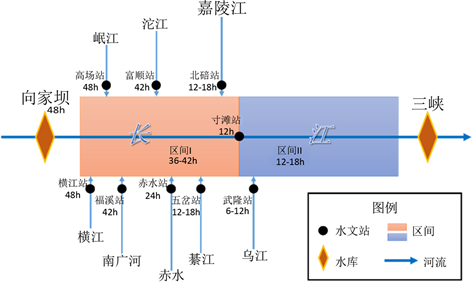Figure 2. Sketch maps and key elements in study area

3.1. 数据输入的优选结果Table 1. Results of optimal input combination

3.2. 水文预报精度分析Table 2. Setting parameters in neural network models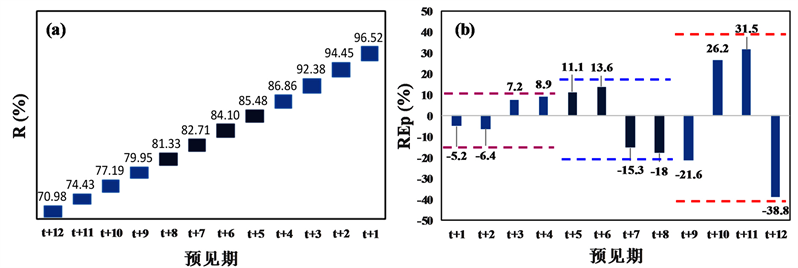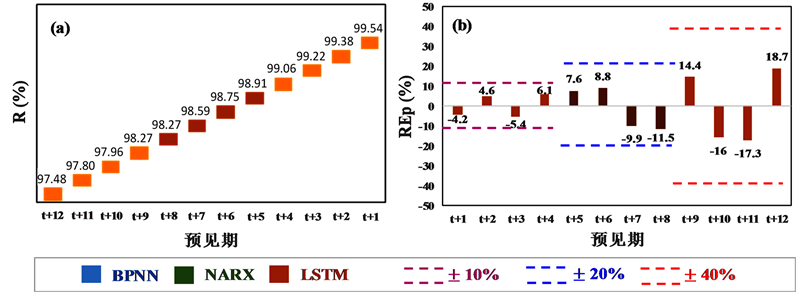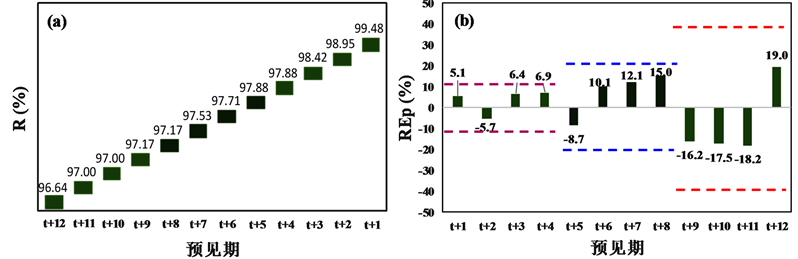Figure 3. Reliability and relative error of peak discharge during testing period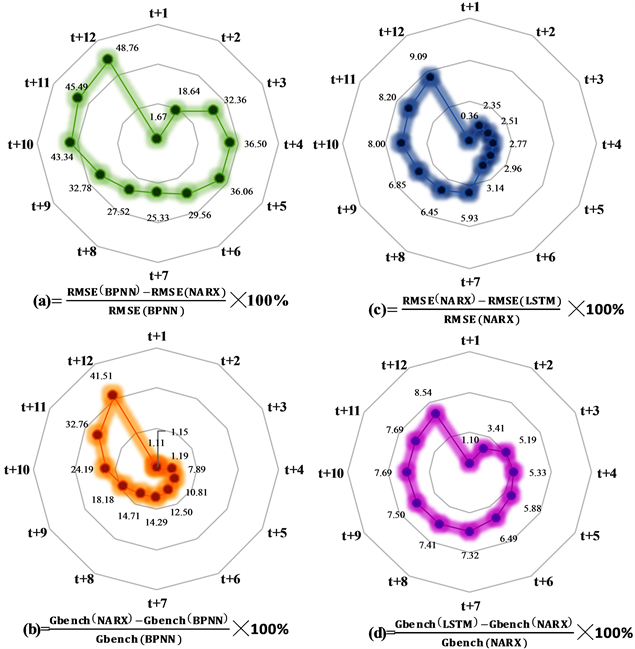Figure 4. Root mean square error and goodness of fit during testing period

4. 结论

 王浩, 李扬, 任立良, 等. 水文模型不确定性及集合模拟总体框架[J]. 水利水电技术, 2015, 46(6): 21-26. WANG Hao, LI Yang, REN Liliang, et al. Uncertainty of hydrologic model and general framework of ensemble simulation. Water Resources and Hydropower Engineering, 2015, 46(6): 21-26. (in Chinese)

 张建云. 中国水文预报技术发展的回顾与思考[J]. 水科学进展, 2010, 21(4): 435-443. ZHANG Jianyun. Review and reflection on China’s hydrological forecasting techniques. Advances in Water Science, 2010, 21(4): 435-443. (in Chinese)

 ABRAHART, R. J., ANCTIL, F., COULIBALY, P., et al. Two decades of anarchy? Emerging themes and outstanding challenges for neural network river forecasting. Progress in Physical Geography, 2012, 36(4): 480-513.
https://doi.org/10.1177/0309133312444943

 DARRAS, T., BORRELL ESTUPINA, V., KONGASIOU, L., et al. Identification of spatial and temporal contributions of rainfalls to flash floods using neural network modeling: Case study on the Lez basin (southern France). Hydrology and Earth System Sciences, 2015, 19(4): 4397-4410.
https://doi.org/10.5194/hess-19-4397-2015

 BAI, Y., CHEN, Z., XIE, J., et al. Daily reservoir inflow forecasting using multiscale deep feature learning with hybrid models. Journal of Hydrology, 2016, 532: 193-206.

 CHANG, F. J., TSAI, M. J. A nonlinear spatio-temporal lumping of radar rainfall for modeling multi-step-ahead inflow forecasts by data-driven techniques. Journal of Hydrology, 2016, 535: 256-269.

 SEO, Y., KIM, S., KISI, O., et al. Daily water level forecasting using wavelet decomposition and artificial intelligence techniques. Journal of Hydrology, 2015, 520(10): 224-243.
https://doi.org/10.1016/j.jhydrol.2014.11.050

 El-SHAFIE, A., NOURELDIN, A., TAHA, M. R., et al. Dynamic versus static neural network model for rainfall forecasting at Klang River Basin, Malaysia. Hydrology and Earth System Sciences, 2011, 16(4): 1151-1169.
https://doi.org/10.5194/hess-16-1151-2012

 GRAVES, A. Supervised sequence labeling with recurrent neural networks. Berlin Heidelberg: Springer, 2012.

 张弛, 王本德, 李伟. 数据挖掘技术在水文预报中的应用及水文预报发展趋势研究[J]. 水文, 2007, 27(2): 74-77. ZAHNG Chi, WANG Bende and LI Wei. Application of data mining technology in hydrological forecasting and research on development trend of hydrological forecasting. Journal of China Hydrology, 2007, 27(2): 74-77. (in Chi-nese)

 STEFÁNSSON, A., KONČAR, N. and JONES, A. J. A note on the Gamma test. Neural Computing and Applications, 1997, 5(3): 131-133.
https://doi.org/10.1007/BF01413858

 任启伟, 陈洋波. 基于Gamma Test的非线性降雨径流回归模型研究[J]. 水文, 2010, 30(1): 39-43. REN Qiwei, CHENG Yangbo. Analysis of nonlinear rainfall-runoff regression modeling based on Gamma test. Journal of China Hydrology, 2010, 30(1): 39-43. (in Chinese)

 LEONTARITIS, I. J. and BILLINGS, S. A. Input-output parametric models for non-linear systems. Part I: Deterministic non-linear systems, Part II: stochastic non-linear systems. International Journal of Control, 1985, 41(2): 323-344.

 HOCHREITER, S. and SCHMIDHUBER, J. Long short-term memory. Neural Computation, 1997, 9(8): 1735-1780.
https://doi.org/10.1162/neco.1997.9.8.1735

 水利部. 水文情报预报规范(GB/T22482-2008) [S]. 北京: 中国标准出版社, 2009. Ministry of Water Resources. Hydrology information forecast specification (GB/T22482-2008). Beijing: China Standards Press, 2009. (in Chinese)

Top